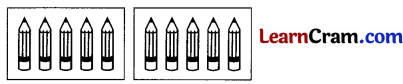# DAV Class 3 Maths Chapter 5 Worksheet 1 Solutions

The DAV Class 3 Maths Book Solutions and DAV Class 3 Maths Chapter 5 Worksheet 1 Solutions of Division offer comprehensive answers to textbook questions.

## DAV Class 3 Maths Ch 5 WS 1 Solutions

Read carefully the statements and fill in the boxes.

Question 1.

There are 15 flowers.
There are 3 flower pots having equal number of flowers.
There are ____ flowers in each pot.
Solution:
There are 5 flowers in each pot.
15 ÷ 3 = 5

Question 2.
There are 20 books.
There are 5 shelves having equal number of books.
There are ____ books in each shelf.
Solution:
There are 4 books in each shelf.
20 ÷ 5 = 4Question 3.
There are 60 handkerchiefs.
There are 6 packets having equal number of handkerchiefs.
There are ____ handkerchief in each packet.
Solution:
There are 10 handkerchief in each packet.
60 ÷ 6 = 10

Question 4.
There are 28 cherries.
There are 4 cakes having equal number of cherries.
There are ____ cherries on each cake.
Solution:
There are 7 cherries on each cake.
28 ÷ 4 = 7### DAV Class 3 Maths Chapter 5 Worksheet 1 Notes

• ÷ is the symbol for division.
• 8 ÷ 8 = 1 The number divided by same no. is equal to 1
• 8 ÷ 1 = 8 The number divided by one is the number itself
• 0 ÷ 7 = 0 0 ÷ counting number = 0

Division by Repeated Subtraction

15 ÷ 5
15 – 5 = 10
10 – 5 = 5
5 – 5 = 0
15 ÷ 5 = 3
(5 can be subtracted 3 times from 15)

Division and Multiplication are RelatedMultiplication fact 2 × 3 = 66 crayons divided into 2 equal groups gives 3 crayons in each.
6 ÷ 2 = 3Let us find how many are there in each group. (See fig Textbook Page 48)

Question 1.
There are 15 flowers.
They are arranged equally in 3 vases.
Solution:
There are 5 flowers in each vase.

Question 2.
There are 12 toffees.
They are in 2 packets.
Solution:
There are 6 toffees in each packet.

Question 3.
There are 10 marbles.
They are in 2 bowls.
Solution:
There are 5 marbles in each bowl.

Question 4.
There are 10 pencils.
Draw equal no. of pencils in two boxes.
Solution:Question 5.
There are 18 leaves.
Draw equal number of leaves in three boxes.
Solution: